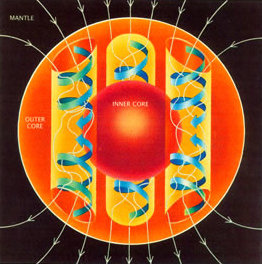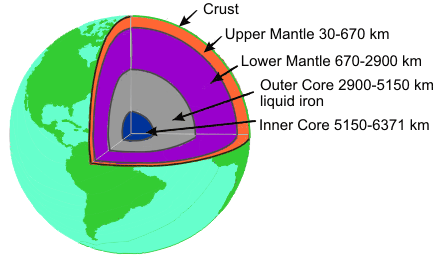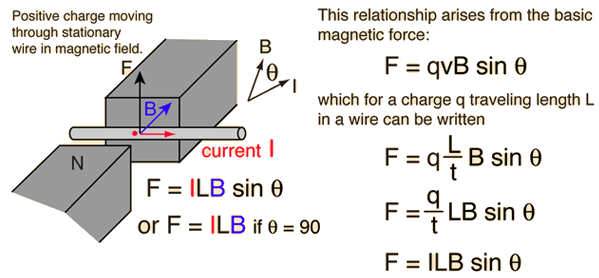Home » Posts tagged 'Magnetism'

# Tag Archives: Magnetism

## Magnetism of Earth – What’s the cause?

Selwyn posted this Message: I was recently showing my grandchildren the effect on a compass needle by the magnetic field surrounding a magnet placed in opposition to the earth’s magnetic field – Magnetism 1.01 no? Their mother then asked me how is it that the earth has a magnetic field. I confidently answered that it was due to the central molten iron core of the earth acting as a magnet, and of course as we learned in Magnetism 1.1 68 years ago on of the ways of creating a magnet is a) by striking it several blows with a hammer while holding it in alignment with the earth’s N/S axis or b) heating it. In both cases this allows the atoms to move more freely and align themselves similarly with the axis. But that started me thinking: We know that heating ANYTHING applies energy to the atoms/molecules and this then causes them to display greater and more violent movement within the body of the material. If these molecules are so agitated, how can this identical phenomenon allow the molecule/atoms of the earth’s core to “settle down quietly” into a N/S configuration and remain so?

The answer is not so simple. Nobody has actually drilled into the centre of earth. What we know is by analysing the seismic waves and the shockwaves.

The Earth’s magnetic field is believed to be generated by electric currents in the conductive material of its core, created by convection currents due to heat escaping from the core. However the process is complex, and computer models that reproduce some of its features have only been developed in the last few decades. (Wikipedia)The Earth and most of the planets in the Solar System, as well as the Sun and other stars, all generate magnetic fields through the motion of highly conductive fluids. The Earth’s field originates in its core. This is a region of iron alloys extending to about 3400 km (the radius of the Earth is 6370 km). It is divided into a solid inner core, with a radius of 1220 km, and a liquid outer core. The motion of the liquid in the outer core is driven by heat flow from the inner core, which is about 6,000 K (5,730 °C; 10,340 °F), to the core-mantle boundary, which is about 3,800 K (3,530 °C; 6,380 °F). The pattern of flow is organized by the rotation of the Earth and the presence of the solid inner core.

The mechanism by which the Earth generates a magnetic field is known as a dynamo.

(Wikipedia)

## The Dynamo Effect

The simple question “how does the Earth get its magnetic field?” does not have a simple answer. It does seem clear that the generation of the magnetic field is linked to the rotation of the earth, since Venus with a similar iron-core composition but a 243 Earth-day rotation period does not have a measurable magnetic field. It certainly seems plausible that it depends upon the rotation of the fluid metallic iron which makes up a large portion of the interior, and the rotating conductor model leads to the term “dynamo effect” or “geodynamo”, evoking the image of an electric generator.Convection drives the outer-core fluid and it circulates relative to the earth. This means the electrically conducting material moves relative to the earth’s magnetic field. If it can obtain a charge by some interaction like friction between layers, an effective current loop could be produced. The magnetic field of a current loop could sustain the magnetic dipole type magnetic field of the earth. Large-scale computer models are approaching a realistic simulation of such a geodynamo.

Refer to this Wikipedia Page and this Hyperphysics page for more details

The GeoMag page is also very informative and useful(Natural Resources Canada)

## Why there are Sine functions in Physics?

Why are sine functions used in some of the equations in physics?
For example,in the expression for instantaneous emf induced in a rotating conductor placed in an uniform magnetic field which is given by
E=B*L*V*sin(theta)
where,
B is the strength of the magnetic field,L is the length of the conductor and (theta) is the angle between the direction of motion of the conductor and the magnetic field,and here,my question is why do we use sine function in this expression?what is its need?The function sin (theta) in a right angled triangle = opp. side /Hypotenuse

When we have to take a component perpendicular to another, we take the sine function.## Magnetism and Matter – The bare essentials for CBSE Physics

You can download the pdf file below to study the magnetism as per CBSE syllabus. This file can also used as TLM (Teaching Learning Material) by teachers to make the classroom teaching more effective and comvinient.

## Force on a current carrying conductor in a magnetic field

Why the force acting on current carrying conductor when placed in a magnetic field increases with the increase in the current.?

I mean what happens inside it at that time? Please explain it in detail, it’s really confusing me, Please answer it as sson as possible.

Let’s consider the terms involved here first.• Electric Current is the rate of flow of charges. An increase in current through a conductor means more number of electrons are crossing any cross section of the wire per second.
• A moving charge experience a force in magnetic field because a moving charge creates a magnetic field around it. The two interacting magnetic fields causes the force.

So, let’s summarize, when there is a current flowing through a conductor placed in a magnetic field, it experiences a force due to the mutual interaction between the magnetic fields – the already existing field and the field produced by the motion of current carriers (free electrons) inside the conductor.

When there is an increase in current, the force of interaction also increase as the numbers of free electrons flowing through any cross section also increases.

## HYSTERISIS

What is hysteresis? I only know its related to the frequency.

Ans:

Hysterisis mens “Lagging Behind”

Hysteresis phenomena occur in magnetic materials, (as well as in the elastic, electric, and magnetic behavior of materials,) in which a lag occurs between the application and the removal of a field (or force) and its subsequent effect

The lack of retraceability of the magnetization curve is the property called hysteresis

#### Magnetic hysteresisWhen an external magnetic field is applied to a ferromagnetic substance, the atomic dipoles align themselves with the external field. Even when the external field is removed, part of the alignment will be retained: the material has become magnetized.

The relationship between magnetic field strength (H)(EXTERNAL) and magnetic flux density (B) (ACQUIRED) is not linear in such materials. If the relationship between the two is plotted for increasing levels of field strength, it will follow a curve up to a point where further increases in magnetic field strength will result in no further change in flux density. This condition is called magnetic saturation.

If the magnetic field is now reduced linearly, the plotted relationship will follow a different curve back towards zero field strength at which point it will be offset from the original curve by an amount called the remanent flux density or remanence (RETENTIVITY).

If this relationship is plotted for all strengths of applied magnetic field the result is a sort of S- shaped loop. (See the diagram) The ‘thickness’ of the middle bit of the S describes the amount of hysteresis, related to the coercivity of the material.

## Magnetism and Laws of motion

“Can a body at rest make another body move,according to the laws of Newton this is impossible. The question is in magnetism, If it happens then why is it not predicted by classical mechanics?”

Answer: First of all I congratulate you for your genuine thought. It is the critical thinking which will lead you to great discoveries.

To answer your question, let me ask you the question – When an apple falls to earth, the earth is also attracted by the apple by an equal and opposite force, then why earth is not moving towards the apple?

The difference lies in the acceleration achieved.  a= F/m

Since mass of earth is very much large, its acceleration towards apple is negligibly small compared to the acceleration due to gravity (9.8 m/s2)

When the magnet attracts another magnet or a magnetic substance, both are experiencing the same force. But it is common that we generally hold the magnet restricting its motion. Therefore, it is not able to move towards the other one.

Newton’s third law is valid and correct even if the forces are contact forces or non contact forces.

## Motion of electron in Electric and Magnetic Fields

“Both magnetic and electric feilds are perpendicular in a region.If we place an electron at rest in that region.what will be the path followed by that electron ?”

When the electron is at rest, it is not affected by magnetic field, but due to electric field, it will begin to move in a direction opposite to the field. This initial direction determines the plane of motion. Then it is acted upon by magnetic field as the motion starts

(The answer is incomplete. I am leaving it for the visitors to think and respond)

Charge is placed at rest in crossed electric and magnetic fields
Let electric and magnetic fields are aligned along z and x directions and charge is placed at the origin of coordinate system. Initially, there is no magnetic force as charge is at rest.However, there is electric force, which accelerates the charge in z-direction. As the particle acquires velocity in z-direction, the magnetic force comes into play and tries to rotate the particle in xz plane about a center on x-axis.
However, z-component of velocity keeps increasing with time due to electric force in that direction. The magnetic force though draws the charged particle away from z-axis along a curved path. This action of magnetic force is countered by electric force in z-direction. The velocity of charged particle ultimately reduces to zero at x-axis. This cycle repeats itself forming cycloid motion. The cycloid path is generated by a point on the circumference of a
rolling wheel.

### Hits so far @ AskPhysics

• 2,171,686 hits

### CBSE Exam Count Down

AISSCE / AISSE 2020March 1, 2019
Board Exams for the core subjects of CBSE may start from 1 March. (Some subjects will have exams in Feb itself)